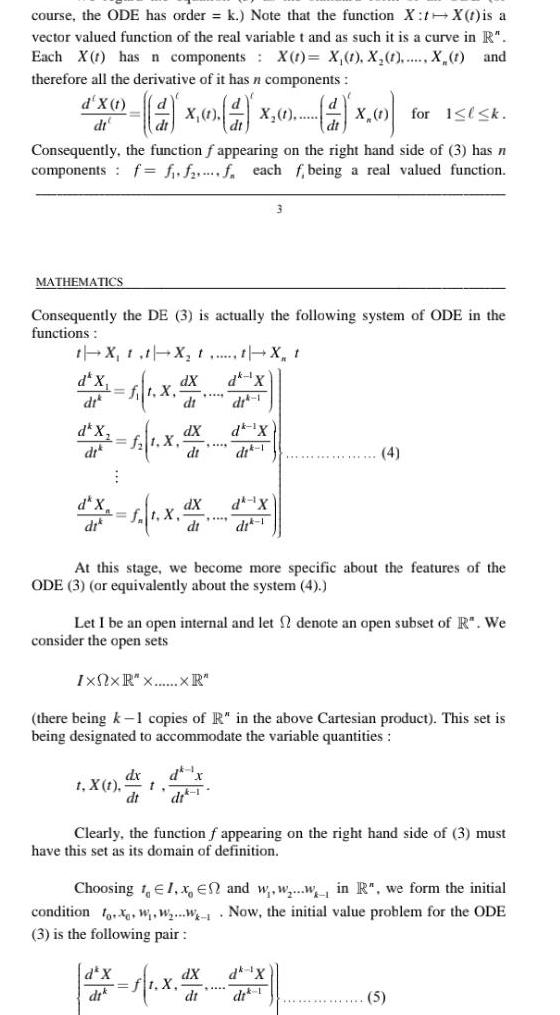Question:

# course the ODE has order k Note that the function X t X t is

Last updated: 9/18/2023course the ODE has order k Note that the function X t X t is a vector valued function of the real variable t and as such it is a curve in R Each X has n components X t X 1 X 1 X t and therefore all the derivative of it has n components X 1 X 1 d for 1 l k Consequently the function f appearing on the right hand side of 3 has n components f f f f each f being a real valued function d X 1 dt MATHEMATICS Consequently the DE 3 is actually the following system of ODE in the functions t X t t X t t X t dk X dit 1 dk 1X d x f X d X di di d X dik t X t 1 X 1 X dx dt d X dt dX dt dX dt t dX At this stage we become more specific about the features of the ODE 3 or equivalently about the system 4 Let I be an open internal and let 2 denote an open subset of R We consider the open sets IxNxR x XR there being k 1 copies of R in the above Cartesian product This set is being designated to accommodate the variable quantities d x dr 1 3 dk X dit 1 1 X Clearly the function f appearing on the right hand side of 3 must have this set as its domain of definition dX dt Choosing 1 1 x En and w w w in R we form the initial condition fox W W W Now the initial value problem for the ODE 3 is the following pair 4 dk X 5